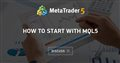# strange valeur of STOP LOSS

hello, I start programming in MQL5, and I progress little by little but I do not understand an event. I put the code below but when I run the Script and look at the log in MT5 it tells me that my stop loss is not good (stop loss = 700,000). At the beginning I thought to myself that I had coded badly so I looked at other EAs that worked well but I did not find any difference with my code which would justify such a value. Do you have an idea ?

```//+------------------------------------------------------------------+
//|                                               Test_programme.mq5 |
//|                                  Copyright 2021, MetaQuotes Ltd. |
//|                                             https://www.mql5.com |
//+------------------------------------------------------------------+
#property version   "1.00"

#include <Expert/Expert.mqh>

input int Stop_Loss=30;
input int Take_Profit=70;
input string Volume_position=0.1;
input int  EA_Magic=1;
CPositionInfo M_position;
CSymbolInfo M_symbol;

int STP;
int TKP;
//+------------------------------------------------------------------+
//|                                                                  |
//+------------------------------------------------------------------+

int OnInit(){
M_symbol.Name(Symbol());

STP = Stop_Loss;
TKP = Take_Profit;
if(M_symbol.Digits()==5 || M_symbol.Digits()==3)
{
STP = STP*10;
TKP = TKP*10;
}
return(0);
}

//+------------------------------------------------------------------+
//|                                                                  |
//+------------------------------------------------------------------+
void OnTick()
{

MqlTick latest_price;
bool Sell_opened=false;

if(PositionSelect(_Symbol)==true) // we have an opened position
{
if(PositionGetInteger(POSITION_MAGIC)==1)
{
{
}
else
if(PositionGetInteger(POSITION_TYPE)==POSITION_TYPE_SELL)
{
Sell_opened=true; // It is a Sell
}
}
}
if(iRSI(_Symbol,PERIOD_CURRENT,14,PRICE_CLOSE)<30)
{
{
return;
}

}
if(iRSI(_Symbol,PERIOD_CURRENT,14,PRICE_CLOSE)>80)
{
if(Sell_opened==true)
{
return;
}

OP_SELL();
}
}

double StopLossLevel=0.0;
double TakeProfitLevel=0.0;

double volume=Volume_position;
if(volume!=0.0)
}

//-----
void OP_SELL(){

double StopLossLevel=0.0;
double TakeProfitLevel=0.0;
StopLossLevel=M_symbol.NormalizePrice(M_symbol.Bid()+TKP);
TakeProfitLevel=M_symbol.NormalizePrice(M_symbol.Bid()-STP);
double volume=Volume_position;
if(volume!=0.0)
}

//+------------------------------------------------------------------+
```

EA opens Many Trades, How to Open 1 buy & 1 sell?

You have a huge mistake - you create an indicator handle at every tick.

REMEMBER: The MQL5 style implies that the indicator handle MUST be created ONLY ONCE and this must be done in OnInit !!!

### An example of get values from the iRSI indicator